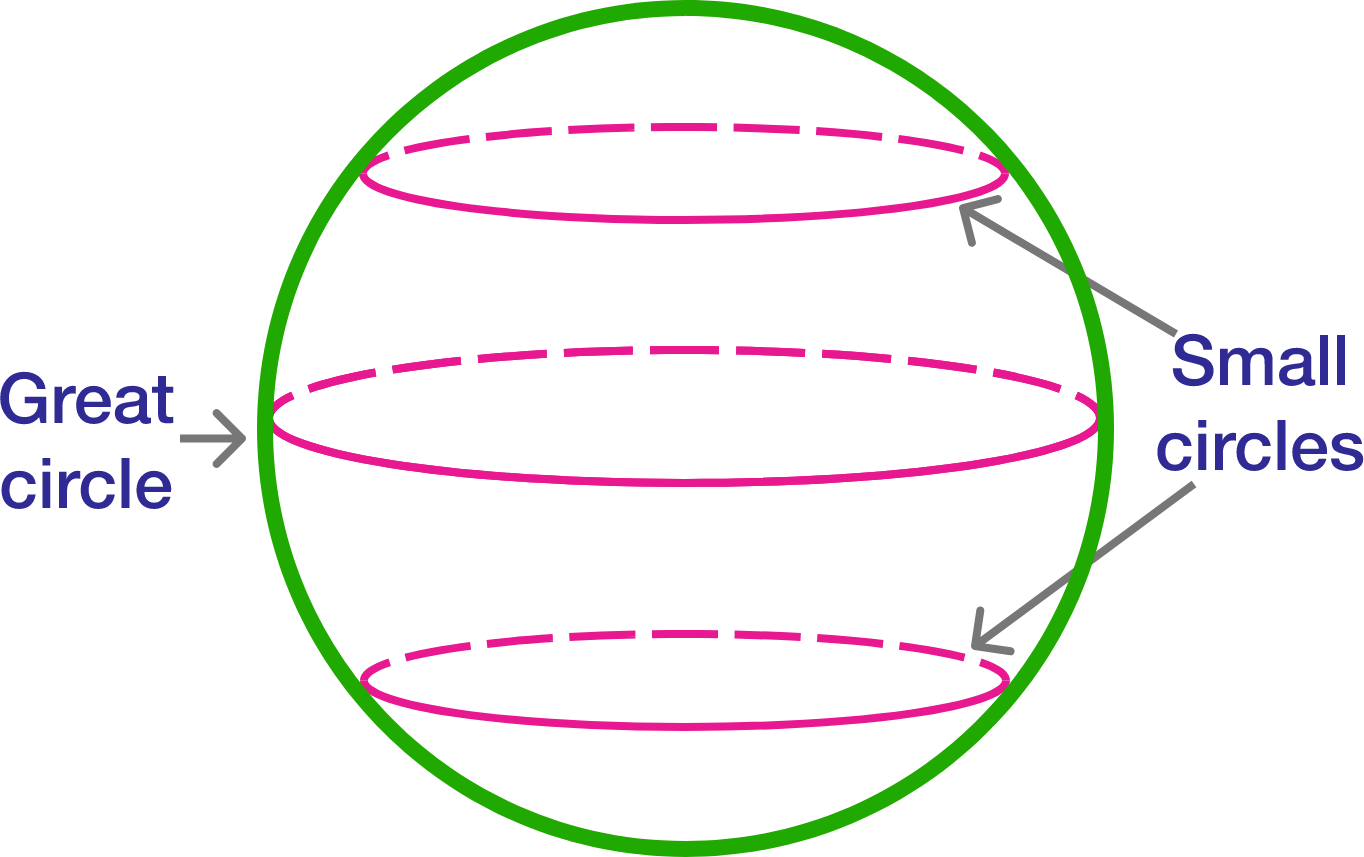# The great circle

Probability Level 4

Given a sphere, a circle is called a great circle if it is the intersection of the sphere with a plane passing through its center.Now, 5 distinct great circles dissect a sphere into $n$ pieces. If $m$ and $M$ are the minimum and maximum values of $n$, respectively, then find $m+M$.

×

Problem Loading...

Note Loading...

Set Loading...